# 一类奇异摄动抛物型反应扩散问题的数值解Numerical Solution of a Class of Singularly Perturbed Parabolic Reaction-Diffusion Problem

DOI: 10.12677/AAM.2019.86136, PDF, HTML, XML, 下载: 320  浏览: 408

Abstract: The singularly perturbed problem’s high accuracy numerical method is always needed. In this paper, barycentric Lagrange interpolation collocation method (BLICM) is proposed for solving a class of singularly perturbed parabolic reaction-diffusion problem. Compared with other methods, the numerical experiment shows BLICM is a high precision method to solve this class of problems.

1. 引言

$\left\{\begin{array}{l}\frac{\partial u}{\partial t}-{\epsilon }_{1}\frac{{\partial }^{2}u}{\partial {x}^{2}}+{\alpha }_{1}u+{\alpha }_{2}v+{\alpha }_{3}r={f}_{1}\left(x,t\right),\\ \frac{\partial v}{\partial t}-{\epsilon }_{2}\frac{{\partial }^{2}v}{\partial {x}^{2}}+{\beta }_{1}u+{\beta }_{2}v+{\beta }_{3}r={f}_{2}\left(x,t\right),\left(x,t\right)\in \left[0,1\right]×\left[0,1\right],\\ \frac{\partial r}{\partial t}-{\epsilon }_{3}\frac{{\partial }^{2}r}{\partial {x}^{2}}+{\gamma }_{1}u+{\gamma }_{2}v+{\gamma }_{3}r={f}_{3}\left(x,t\right),\end{array}$ (1)

$\begin{array}{l}u\left(x,0\right)={f}_{0}\left(x\right),v\left(x,0\right)={g}_{0}\left(x\right),r\left(x,0\right)={s}_{0}\left(x\right),x\in \left[0,1\right]\\ u\left(0,t\right)={f}_{1}\left(t\right),u\left(1,t\right)={f}_{2}\left(t\right),\\ v\left(0,t\right)={g}_{1}\left(t\right),v\left(1,t\right)={g}_{2}\left(t\right),t\in \left[0,1\right]\\ r\left(0,t\right)={s}_{1}\left(t\right),r\left(1,t\right)={s}_{2}\left(t\right),\end{array}$ (2)

2. BLICM的描述

$\begin{array}{l}{x}_{i}=-\mathrm{cos}\left(\frac{i-1}{M-1}\right)\text{π},i=1,2,\cdots ,M,\\ {t}_{j}=-\mathrm{cos}\left(\frac{j-1}{N-1}\right)\text{π},j=1,2,\cdots ,N.\end{array}$ (3)

$\begin{array}{l}u\left(x,t\right)=\underset{i=1}{\overset{M}{\sum }}\underset{j=1}{\overset{N}{\sum }}{\xi }_{i}\left(x\right){\eta }_{j}\left(t\right)u\left({x}_{i},{t}_{j}\right),\\ v\left(x,t\right)=\underset{i=1}{\overset{M}{\sum }}\underset{j=1}{\overset{N}{\sum }}{\xi }_{i}\left(x\right){\eta }_{j}\left(t\right)v\left({x}_{i},{t}_{j}\right),\\ r\left(x,t\right)=\underset{i=1}{\overset{M}{\sum }}\underset{j=1}{\overset{N}{\sum }}{\xi }_{i}\left(x\right){\eta }_{j}\left(t\right)r\left({x}_{i},{t}_{j}\right),\end{array}$ (4)

$\begin{array}{l}\frac{{\partial }^{l+k}u}{\partial {x}^{l}\partial {t}^{k}}=\underset{i=1}{\overset{M}{\sum }}\underset{j=1}{\overset{N}{\sum }}{\xi }_{i}^{\left(l\right)}\left(x\right){\eta }_{j}^{\left(k\right)}\left(t\right)u\left({x}_{i},{t}_{j}\right),l,k=1,2,\cdots ,\\ \frac{{\partial }^{l+k}v}{\partial {x}^{l}\partial {t}^{k}}=\underset{i=1}{\overset{M}{\sum }}\underset{j=1}{\overset{N}{\sum }}{\xi }_{i}^{\left(l\right)}\left(x\right){\eta }_{j}^{\left(k\right)}\left(t\right)v\left({x}_{i},{t}_{j}\right),l,k=1,2,\cdots ,\\ \frac{{\partial }^{l+k}r}{\partial {x}^{l}\partial {t}^{k}}=\underset{i=1}{\overset{M}{\sum }}\underset{j=1}{\overset{N}{\sum }}{\xi }_{i}^{\left(l\right)}\left(x\right){\eta }_{j}^{\left(k\right)}\left(t\right)r\left({x}_{i},{t}_{j}\right),l,k=1,2,\cdots ,\end{array}$ (5)

$\begin{array}{l}{u}^{\left(l,k\right)}\left({x}_{p},{t}_{q}\right)=\frac{{\partial }^{l+k}u\left({x}_{p},{t}_{q}\right)}{\partial {x}^{l}\partial {t}^{k}}=\underset{i=1}{\overset{M}{\sum }}\underset{j=1}{\overset{N}{\sum }}{\xi }_{i}^{\left(l\right)}\left({x}_{p}\right){\eta }_{j}^{\left(k\right)}\left({t}_{q}\right)u\left({x}_{p},{t}_{q}\right),p=1,2,\cdots ,M;q=1,2,\cdots ,N,\\ {v}^{\left(l,k\right)}\left({x}_{p},{t}_{q}\right)=\frac{{\partial }^{l+k}v\left({x}_{p},{t}_{q}\right)}{\partial {x}^{l}\partial {t}^{k}}=\underset{i=1}{\overset{M}{\sum }}\underset{j=1}{\overset{N}{\sum }}{\xi }_{i}^{\left(l\right)}\left({x}_{p}\right){\eta }_{j}^{\left(k\right)}\left({t}_{q}\right)v\left({x}_{p},{t}_{q}\right),p=1,2,\cdots ,M;q=1,2,\cdots ,N,\\ {r}^{\left(l,k\right)}\left({x}_{p},t{}_{q}\right)=\frac{{\partial }^{l+k}r\left({x}_{p},{t}_{q}\right)}{\partial {x}^{l}\partial {t}^{k}}=\underset{i=1}{\overset{M}{\sum }}\underset{j=1}{\overset{N}{\sum }}{\xi }_{i}^{\left(l\right)}\left({x}_{p}\right){\eta }_{j}^{\left(k\right)}\left({t}_{q}\right)r\left({x}_{p},{t}_{q}\right),p=1,2,\cdots ,M;q=1,2,\cdots ,N.\end{array}$ (6)

${x}^{0}={\left[{x}_{1},{x}_{2},\cdots ,{x}_{M}\right]}^{\text{T}}$${t}^{0}={\left[{t}_{1},{t}_{2},\cdots ,{t}_{N}\right]}^{\text{T}}$ 分别定义为x轴和t轴，由张量型节点坐标组成的矩阵X和T

$X={\left[{\left({x}^{0}\right)}^{\text{T}},{\left({x}^{0}\right)}^{\text{T}},\cdots ,{\left({x}^{0}\right)}^{\text{T}}\right]}^{\text{T}},T=\left[{t}^{0},{t}^{0},\cdots ,{t}^{0}\right].$ (7)

$x,t$ 是由矩阵 $X,T$ 拉伸的 $\left(N×M\right)$ 维列向量：

$x={\left[{X}_{1},{X}_{2},\cdots ,{X}_{M×N}\right]}^{\text{T}},\text{\hspace{0.17em}}t={\left[{T}_{1},{T}_{2},\cdots ,{T}_{M×N}\right]}^{\text{T}}.$ (8)

${X}_{k}={X}_{\left(i-1\right)N+j}={x}_{i},\text{\hspace{0.17em}}{T}_{k}={T}_{\left(i-1\right)N+j}={t}_{j},\text{\hspace{0.17em}}i=1,2,\cdots ,M;J=1,2,\cdots ,N;k=1,2,\cdots ,M×N.$ (9)

$u={\left[{u}_{1},{u}_{2},\cdots ,{u}_{M×N}\right]}^{\text{T}},\text{\hspace{0.17em}}\text{\hspace{0.17em}}{u}^{\left(l,k\right)}={\left[{u}_{1}^{\left(l,k\right)},{u}_{2}^{\left(l,k\right)},\cdots ,{u}_{M×N}^{\left(l,k\right)}\right]}^{\text{T}},$

${u}_{p}=u\left({X}_{p},{T}_{p}\right),\text{\hspace{0.17em}}\text{\hspace{0.17em}}{u}_{p}^{\left(l,k\right)}={u}^{\left(l,k\right)}\left({X}_{p},{T}_{p}\right),\text{\hspace{0.17em}}p=1,2,\cdots ,M×N.$

$v={\left[{v}_{1},{v}_{2},\cdots ,{v}_{M×N}\right]}^{\text{T}},\text{\hspace{0.17em}}\text{\hspace{0.17em}}{v}^{\left(l,k\right)}={\left[{v}_{1}^{\left(l,k\right)},{v}_{2}^{\left(l,k\right)},\cdots ,{v}_{M×N}^{\left(l,k\right)}\right]}^{\text{T}},$

${v}_{p}=v\left({X}_{p},{T}_{p}\right),\text{\hspace{0.17em}}\text{\hspace{0.17em}}{v}_{p}^{\left(l,k\right)}={v}^{\left(l,k\right)}\left({X}_{p},{T}_{p}\right),\text{\hspace{0.17em}}p=1,2,\cdots ,M×N.$

$r={\left[{r}_{1},{r}_{2},\cdots ,{r}_{M×N}\right]}^{\text{T}},\text{\hspace{0.17em}}\text{\hspace{0.17em}}{r}_{p}^{\left(l,k\right)}={\left[{r}_{1}^{\left(l,k\right)},{r}_{2}^{\left(l,k\right)},\cdots ,{r}_{M×N}^{\left(l,k\right)}\right]}^{\text{T}},$

${r}_{p}=r\left({X}_{p},{T}_{p}\right),\text{\hspace{0.17em}}\text{\hspace{0.17em}}{r}_{p}^{\left(l,k\right)}={r}^{\left(l,k\right)}\left({X}_{p},{T}_{p}\right),\text{\hspace{0.17em}}p=1,2,\cdots ,M×N.$

${u}^{\left(l,k\right)}={D}^{\left(l,k\right)}u,\text{\hspace{0.17em}}\text{\hspace{0.17em}}{v}^{\left(l,k\right)}={D}^{\left(l,k\right)}v,\text{\hspace{0.17em}}\text{\hspace{0.17em}}{r}^{\left(l,k\right)}={D}^{\left(l,k\right)}r.$ (10)

$\left[\begin{array}{ccc}{D}^{\left(0,1\right)}-{\epsilon }_{1}{D}^{\left(2,0\right)}+{\alpha }_{1}I& {\alpha }_{2}I& {\alpha }_{3}I\\ {\beta }_{1}I& {D}^{\left(0,1\right)}-{\epsilon }_{2}{D}^{\left(2,0\right)}+{\beta }_{2}I& {\beta }_{3}I\\ {\gamma }_{1}I& {\gamma }_{2}I& {D}^{\left(0,1\right)}-{\epsilon }_{3}{D}^{\left(2,0\right)}+{\gamma }_{3}I\end{array}\right]\left[\begin{array}{l}u\\ v\\ r\end{array}\right]=\left[\begin{array}{l}{f}_{1}\\ {f}_{2}\\ {f}_{3}\end{array}\right]$ (11)

$\begin{array}{l}\underset{i=1}{\overset{M}{\sum }}\underset{j=1}{\overset{N}{\sum }}{\xi }_{i}\left({x}_{p}\right){\eta }_{j}\left(0\right){u}_{ij}={f}_{0}\left({x}_{p}\right),\\ \underset{i=1}{\overset{M}{\sum }}\underset{j=1}{\overset{N}{\sum }}{{\xi }^{\prime }}_{i}\left({x}_{p}\right){{\eta }^{\prime }}_{j}\left(0\right){v}_{ij}={g}_{0}\left({x}_{p}\right),\\ \underset{i=1}{\overset{M}{\sum }}\underset{j=1}{\overset{N}{\sum }}{{\xi }^{″}}_{i}\left({x}_{p}\right){{\eta }^{″}}_{j}\left(0\right){r}_{ij}={s}_{0}\left({x}_{p}\right),\end{array}$ $\begin{array}{l}\underset{i=1}{\overset{M}{\sum }}\underset{j=1}{\overset{N}{\sum }}{\xi }_{i}\left(0\right){\eta }_{j}\left({t}_{q}\right){u}_{ij}={f}_{1}\left({t}_{q}\right),\\ \underset{i=1}{\overset{M}{\sum }}\underset{j=1}{\overset{N}{\sum }}{{\xi }^{\prime }}_{i}\left(0\right){{\eta }^{\prime }}_{j}\left({t}_{q}\right){v}_{ij}={g}_{1}\left({t}_{q}\right),\\ \underset{i=1}{\overset{M}{\sum }}\underset{j=1}{\overset{N}{\sum }}{{\xi }^{″}}_{i}\left(0\right){{\eta }^{″}}_{j}\left({t}_{q}\right){r}_{ij}={s}_{1}\left({t}_{q}\right),\end{array}$ $\begin{array}{l}\underset{i=1}{\overset{M}{\sum }}\underset{j=1}{\overset{N}{\sum }}{\xi }_{i}\left(1\right){\eta }_{j}\left({t}_{q}\right){u}_{ij}={f}_{2}\left({t}_{q}\right),\\ \underset{i=1}{\overset{M}{\sum }}\underset{j=1}{\overset{N}{\sum }}{\xi }_{i}{}^{\prime }\left(1\right){{\eta }^{\prime }}_{j}\left({t}_{q}\right){v}_{ij}={g}_{2}\left({t}_{q}\right),\\ \underset{i=1}{\overset{M}{\sum }}\underset{j=1}{\overset{N}{\sum }}{{\xi }^{″}}_{i}\left(1\right){{\eta }^{″}}_{j}\left({t}_{q}\right){r}_{ij}={s}_{2}\left({t}_{q}\right),\end{array}$ (12)

3. 数值算例

$\left\{\begin{array}{l}\frac{\partial u}{\partial t}-{\epsilon }_{1}\frac{{\partial }^{2}u}{\partial {x}^{2}}+5u-tv={f}_{1}\left(x,t\right),\\ \frac{\partial v}{\partial t}-{\epsilon }_{2}\frac{{\partial }^{2}v}{\partial {x}^{2}}-tu+5\left(1+xt\right)v-r={f}_{2}\left(x,t\right),\\ \frac{\partial r}{\partial t}-{\epsilon }_{3}\frac{{\partial }^{2}r}{\partial {x}^{2}}-2u-\left(1+{x}^{2}\right)v+\left(7+xt\right)r={f}_{3}\left(x,t\right),\end{array}$ (13)

$u\left(0,t\right)=v\left(0,t\right)=r\left(0,t\right)=0,u\left(1,t\right)=v\left(1,t\right)=r\left(1,t\right)=0,u\left(x,0\right)=v\left(x,0\right)=r\left(x,0\right)=0,$ (14)

${f}_{1}\left(x,t\right)=\left\{\begin{array}{l}2+x+t,\text{\hspace{0.17em}}\text{\hspace{0.17em}}\text{\hspace{0.17em}}\text{\hspace{0.17em}}\text{\hspace{0.17em}}\text{\hspace{0.17em}}\text{\hspace{0.17em}}0\le x\le 0.5,\\ 1,\text{\hspace{0.17em}}\text{\hspace{0.17em}}\text{\hspace{0.17em}}\text{\hspace{0.17em}}\text{\hspace{0.17em}}\text{\hspace{0.17em}}\text{\hspace{0.17em}}\text{\hspace{0.17em}}\text{\hspace{0.17em}}\text{\hspace{0.17em}}\text{\hspace{0.17em}}\text{\hspace{0.17em}}\text{\hspace{0.17em}}\text{\hspace{0.17em}}\text{\hspace{0.17em}}\text{\hspace{0.17em}}\text{ }0.5

${f}_{2}\left(x,t\right)=\left\{\begin{array}{l}2\text{\hspace{0.17em}}\text{\hspace{0.17em}}\text{\hspace{0.17em}}\text{\hspace{0.17em}}\text{\hspace{0.17em}}\text{\hspace{0.17em}}\text{\hspace{0.17em}}\text{\hspace{0.17em}}\text{ }0\le x\le 0.5,\\ t,\text{\hspace{0.17em}}\text{\hspace{0.17em}}\text{\hspace{0.17em}}\text{\hspace{0.17em}}\text{\hspace{0.17em}}\text{\hspace{0.17em}}\text{\hspace{0.17em}}\text{\hspace{0.17em}}0.5 (15)

${f}_{3}\left(x,t\right)=\left\{\begin{array}{l}1+x,\text{\hspace{0.17em}}\text{\hspace{0.17em}}\text{\hspace{0.17em}}\text{\hspace{0.17em}}\text{\hspace{0.17em}}\text{\hspace{0.17em}}\text{ }\text{\hspace{0.17em}}\text{\hspace{0.17em}}0\le x\le 0.5,\\ {x}^{2}+t,\text{\hspace{0.17em}}\text{\hspace{0.17em}}\text{\hspace{0.17em}}\text{\hspace{0.17em}}\text{\hspace{0.17em}}\text{\hspace{0.17em}}\text{\hspace{0.17em}}0.5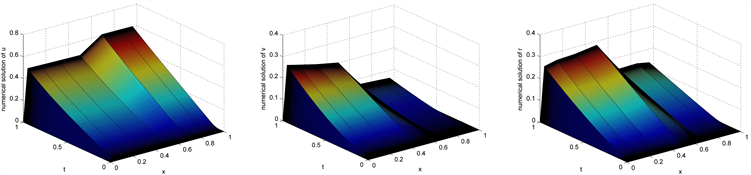Figure 1. Surface plot of the Example 1 for ${\epsilon }_{1}={10}^{-9},{\epsilon }_{2}={10}^{-5},{\epsilon }_{3}={10}^{-3}$ and $N=256,M=8$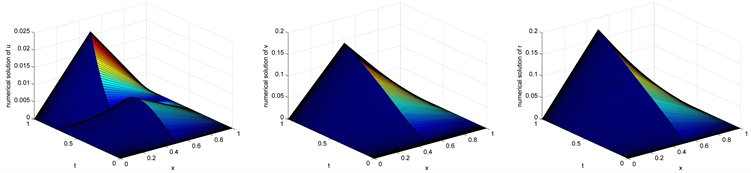Figure 2. Surface plot of the Example 1 for ${\epsilon }_{1}={10}^{-9},{\epsilon }_{2}={10}^{-5},{\epsilon }_{3}={10}^{-3}$ and $N=128,M=2$

Table 1. Comparison of maximum point-wise errors and ε 1 , ε 2 , ε 3 uniform rate of convergence for the example 1Table 2. Comparison of maximum point-wise errors and ε 1 , ε 2 , ε 3 uniform rate of convergence for the Example 1Table 3. Number of iterations required by the algorithm for Example 1

$\left\{\begin{array}{l}\frac{\partial u}{\partial t}-{\epsilon }_{1}\frac{{\partial }^{2}u}{\partial {x}^{2}}+2.1u-\left(1-x\right)v-\left(1+x\right)r=16{x}^{2}{\left(1-x\right)}^{2},\\ \frac{\partial v}{\partial t}-{\epsilon }_{2}\frac{{\partial }^{2}v}{\partial {x}^{2}}-xu+\left(1.1+x\right)v-xr={t}^{2},\\ \frac{\partial r}{\partial t}-{\epsilon }_{3}\frac{{\partial }^{2}r}{\partial {x}^{2}}-\left(2+x\right)u-\left(1-x\right)v-\left(3.1+x\right)r=-16{x}^{2}{\left(1-x\right)}^{2},\end{array}$ (16)

$u\left(0,t\right)=v\left(0,t\right)=r\left(0,t\right)=0,\text{\hspace{0.17em}}\text{\hspace{0.17em}}u\left(1,t\right)=v\left(1,t\right)=r\left(1,t\right)=0.$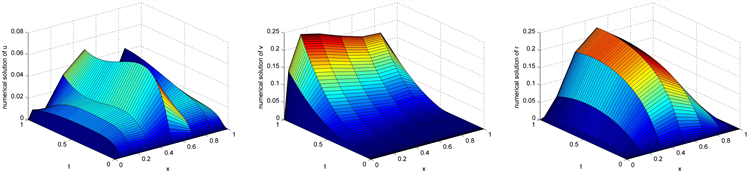Figure 3. Numerical solution of Example 2 for ${\epsilon }_{1}={10}^{-1},{\epsilon }_{2}={10}^{-2},{\epsilon }_{3}={10}^{-3}$ and $N=64,M=8$Table 4. Comparison of maximum point-wise errors and convergence rate for the Example 2

$\left\{\begin{array}{l}\frac{\partial u}{\partial t}-{\epsilon }_{1}\frac{{\partial }^{2}u}{\partial {x}^{2}}+\left(5+xt\right)u-\left({x}^{2}+{t}^{2}\right)v-\left(2+xt\right)r=2\left(1-{\text{e}}^{-t}\right)+5t\mathrm{cos}\left(xt\right),\\ \frac{\partial v}{\partial t}-{\epsilon }_{2}\frac{{\partial }^{2}v}{\partial {x}^{2}}-5xt{\text{e}}^{-x}u+\left(6+\mathrm{cos}\left(xt\right)\right)v-\mathrm{cos}\left(xt\right)r=\left(10x+1\right)t\left({x}^{2}+{t}^{2}-5\mathrm{sin}\left(xt\right)\right),\\ \frac{\partial r}{\partial t}-{\epsilon }_{3}\frac{{\partial }^{2}r}{\partial {x}^{2}}-3x{\text{e}}^{-t}u-\left(xt+\mathrm{sin}\left(x+t\right)\right)v+\left(5+\left(x+1\right){\text{e}}^{-xt}\right)r=-3t\mathrm{cos}\left(x+t\right).\\ u\left(0,t\right)=u\left(1,t\right)=0,t\in \left[0,1\right],u\left(x,0\right)=0,x\in \left[0,1\right].\end{array}$ (17)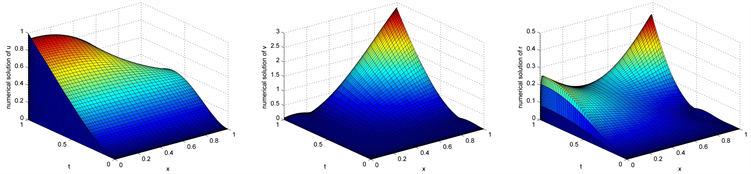Figure 4. The numerical solution for Example 3 at ${\epsilon }_{1}={10}^{-8},{\epsilon }_{2}={10}^{-6},{\epsilon }_{3}={10}^{-4}$Table 5. Comparison of maximum errors and orders of convergence of Example 3Table 6. Comparison of CPU time for Example 3

$\left\{\begin{array}{l}\frac{\partial u}{\partial t}-{\epsilon }_{1}\frac{{\partial }^{2}u}{\partial {x}^{2}}+\left(1+0.5xy\right)\frac{\partial u}{\partial x}+{\text{e}}^{{x}^{2}y}\frac{\partial u}{\partial y}+{\text{e}}^{x+y}\left(1+t\right)u-t\left(x+y\right)v-txr=10{t}^{2}\mathrm{sin}\left(x+y\right),\\ \frac{\partial v}{\partial t}-{\epsilon }_{2}\frac{{\partial }^{2}v}{\partial {x}^{2}}+\left(5+{x}^{2}y\right)\frac{\partial u}{\partial x}+\left(3+\mathrm{sin}\left(x+y\right)\right)\frac{\partial u}{\partial y}-\left(x+y\right)u+\left(1+t\right)\left(3+x+y\right)v-t\mathrm{sin}\left(y\right)r=-5\left(1-{\text{e}}^{-t}\right)\left({x}^{2}+{y}^{2}\right),\\ \frac{\partial r}{\partial t}-{\epsilon }_{3}\frac{{\partial }^{2}r}{\partial {x}^{2}}+\left(3-xy\right)\frac{\partial u}{\partial x}+\left(1+x+y\right)\frac{\partial u}{\partial y}-x{y}^{2}u-t\left(\mathrm{sin}x+\mathrm{sin}y\right)v+{\text{e}}^{t}\left(2+\mathrm{cos}\left(x+y\right)\right)r=-4t{\text{e}}^{t}\mathrm{cos}\left(xy\right),\end{array}$

$\begin{array}{l}u\left(0,y,t\right)=4y\mathrm{sin}\left(t\right),\\ u\left(x,0,t\right)=4x\mathrm{sin}\left(t\right),\end{array}$ $\begin{array}{l}v\left(0,y,t\right)=0,\\ v\left(x,0,t\right)=0,\end{array}$ $\begin{array}{l}r\left(0,y,t\right)=3\left(1-{\text{e}}^{t}\right),\\ r\left(x,0,t\right)=3\left(1-{\text{e}}^{t}\right),\end{array}$

$u\left(x,y,0\right)=v\left(x,y,0\right)=r\left(x,y,0\right)=0,$

$\begin{array}{l}u\left(1,y,t\right)=4\left(1+y\right)\mathrm{sin}\left(t\right),\\ u\left(x,1,t\right)=4\left(x+1\right)\mathrm{sin}\left(t\right),\end{array}$ $\begin{array}{l}v\left(1,y,t\right)=y{t}^{2},\\ v\left(x,1,t\right)=x{t}^{2},\end{array}$ $\begin{array}{l}r\left(1,y,t\right)=3{\text{e}}^{y}\left(1-{\text{e}}^{t}\right),\\ r\left(x,1,t\right)=3{\text{e}}^{x}\left(1-{\text{e}}^{t}\right).\end{array}$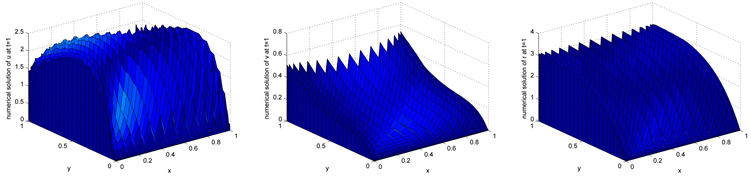Figure 5. Numerical solution of Example 4 at T = 1 for $\epsilon ={10}^{-4}$ and $N=32,M=32$Table 7. Comparison of maximum errors and orders of convergence in Example 4 for v

4. 结论

  Shishkin, G.I. (1989) Approximation of Solutions of Singularly Perturbed Boundary Value Problems with a Parabolic Boundary Layer. Computational Mathematics and Mathematical Physics, 29, 1-10. https://doi.org/10.1016/0041-5553(89)90109-2  Shishkin, G.I. (1995) Mesh Approximation of Singularly Perturbed Boundary Value Problems for Systems of Elliptical and Parabolic Equations. Computational Mathematics and Mathematical Physics, 35, 429-446.  Franklin, V., Paramasivam, M., et al. (2013) Second Order Parame-ter-Uniform Convergence for a Finite Difference Method for a Singularly Perturbed Linear Parabolic System. Interna-tional Journal of Numerical Analysis and Modeling, 10, 178-202.  Gracia, J.L., Lisbona, F.J. and O’Riordan, E. (2010) A Coupled System of Singularly Perturbed Parabolic Reaction-Diffusion Equations. Advances in Computational Mathematics, 32, 43-61. https://doi.org/10.1007/s10444-008-9086-3  Kumar, M. and Rao, S.C.S. (2010) High Order Parameter-Robust Numerical Method for Time Dependent Singularly Perturbed Reaction-Diffusion Problems. Computing, 90, 15-38. https://doi.org/10.1007/s00607-010-0104-1  Linss, T. and Madden, N. (2007) Parameter-Uniform Approximations for Time-Dependent Reaction-Diffusion Problems. Numerical Methods for Partial Differential Equa-tions, 23, 1290-1300. https://doi.org/10.1002/num.20220  MacMullen, H., Miller, J.J.H., O’Riordan, E. and Shishkin, G.I. (2001) A Second-Order Parameter-Uniform Overlapping Schwarz Method for Reaction-Diffusion Prob-lems with Boundary Layers. Journal of Computational and Applied Mathematics, 130, 231-244. https://doi.org/10.1016/S0377-0427(99)00380-5  Chandra Sekhara Rao, S. and Chawla, S. (2018) Numerical Solution of Singularly Perturbed Linear Parabolic System with Discontinuous Source Term. Applied Numerical Mathematics, 127, 249-265. https://doi.org/10.1016/j.apnum.2018.01.006  Kumar, S. and Chandra Sekhara Rao, S. (2014) A Robust Overlapping Schwarz Domain Decomposition Algorithm for Time-Dependent Singularly Perturbed Reaction-Diffusion Problems. Journal of Computational and Applied Mathematics, 261, 127-138. https://doi.org/10.1016/j.cam.2013.10.053  Clavero, C. and Jorge, J.C. (2019) An Efficient Numerical Method for Singularly Perturbed Time Dependent Parabolic 2D Convection—Diffusion Systems. Journal of Computational and Applied Mathematics, 354, 431-444.  Schneiaer, C. and Werner, W. (1986) Some New Aspects of Rational Interpolation. Mathematics of Computation, 175, 285-299. https://doi.org/10.2307/2008095  Berrut, J.P. (1984) Baryzentrische Formeln zur trigonometrischen Interpolation (I). Zeitschrift fur angewandte Mathematik und Physik, 35, 91-105. https://doi.org/10.1007/BF00945179  Berrut, J.P. (1988) Rational Functions for Guaranteed and Experimentally Well Conditioned Global Interpolation. Computers & Mathematics with Applications, 15, 1-16. https://doi.org/10.1016/0898-1221(88)90067-3  Berrut, J.P. and Trefethen, L.N. (2004) Barycentric Lagrange Interpolation. SIAM Review, 46, 501-517. https://doi.org/10.1137/S0036144502417715  Higham, N.J. (2004) The Numerical Stability of Barycentric Lagrange Interpolation. IMA Journal of Numerical Analysis, 24, 547-556. https://doi.org/10.1093/imanum/24.4.547  Mascarenhas, W.F. (2014) The Stability of Barycentric Interpolation at the Chebyshev Points of the Second Kind. Numerische Mathematik, 128, 265-300. https://doi.org/10.1007/s00211-014-0612-6  Li, S.P. and Wang, Z.Q. (2015) Barycentric Interpolation Collo-cation Method for Nonlinear Problems. National Defense Industry Press, Beijing.  Li, S.P. and Wang, Z.Q. (2012) High-Precision Non-Grid Center of Gravity Interpolation Collocation Method: Algorithm, Program and Engineering Application. Science Press, Beijing.  Wang, Y.L., Tian, D. and Li, Z.Y. (2017) Numerical Method for Singularly Perturbed Delay Parabolic Partial Differentiation Equations. Thermal Science, 21, 1595-1599. https://doi.org/10.2298/TSCI160615040W  Liu, F.F., Wang, Y.L. and Li, S.G. (2018) Barycentric Interpola-tion Collocation Method for Solving the Coupled Viscous Burgers’ Equations. International Journal of Computer Mathematics, 95, 2162-2173. https://doi.org/10.1080/00207160.2017.1384546  Wu, H.C., Wang, Y.L. and Zhang, W. (2018) Numerical Solution of a Class of Nonlinear Partial Differential Equations by Using Barycentric Interpolation Collocation Method. Mathematical Problems in Engineering, 2018, Article ID: 7260346. https://doi.org/10.1155/2018/7260346  Wu, H.C., Wang, Y.L., Zhang, W. and Wen, T. (2019) The Barycentric Interpolation Collocation Method for a Class of Nonlinear Vibration Systems. Journal of Low Frequency Noise, Vibration and Active Control, 1-10. https://doi.org/10.1177/1461348418824898  Zhou, X.F., Li, J.M., Wang, Y.L. and Zhang, W. (2019) Nu-merical Simulation of a Class of Hyperchaotic System Using Barycentric Lagrange Interpolation Collocation Method. Complexity, 2019, Article ID: 1739785. https://doi.org/10.1155/2019/1739785  Li, J., Wang, Y.L. and Zhang, W. (2019) Numerical Simulation of the Lorenz-Type Chaotic System Using Barycentric Lagrange Interpolation Collocation Method. Advances in Mathematical Physics, 2019, Article ID: 1030318. https://doi.org/10.1155/2019/1030318  Liu, H.Y., Huang, J., Pan, Y.B. and Zhang, J.P. (2018) Barycentric Interpolation Collocation Methods for Solving Linear and Nonlinear High-Dimensional Fredholm Integral Equations. Computers Mathematics with Applications, 327, Article ID: 141154. https://doi.org/10.1016/j.cam.2017.06.004  Luo, W.H., Huang, T.Z., Gu, X.M. and Liu, Y. (2017) Barycentric Rational Collocation Methods for a Class of Nonlinear Parabolic Partial Differential Equations. Applied Mathematics Letter, 68, 13-19. https://doi.org/10.1016/j.aml.2016.12.011